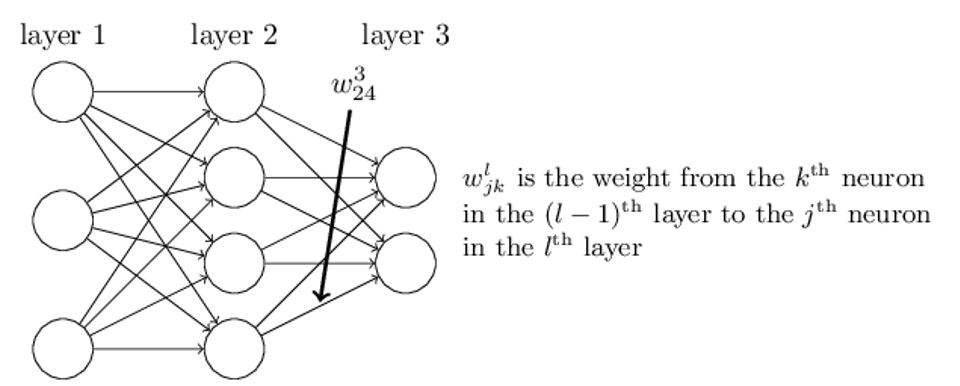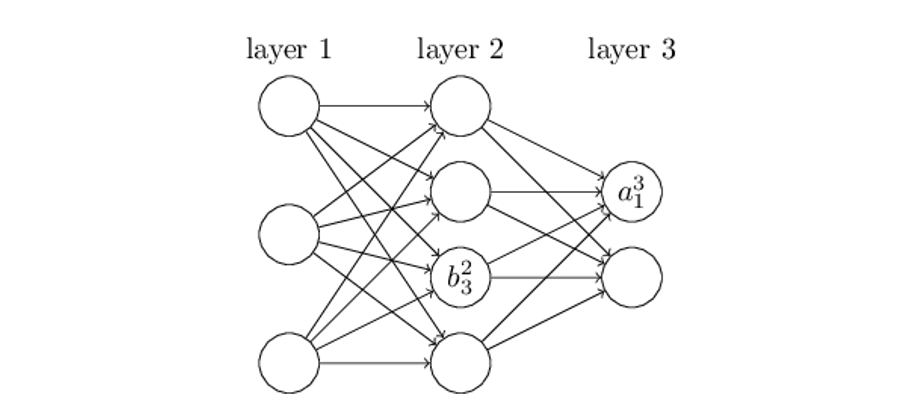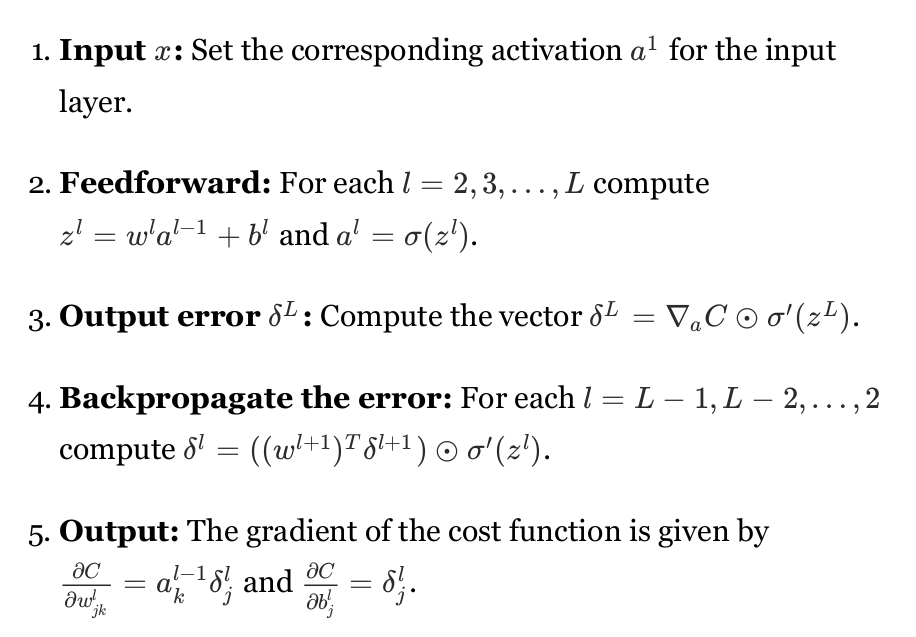## ch-2 How the backpropagation algorithm works

discuss how to compute the gradient ( $\nabla C$ ) of the cost function

Heart of backpropagation

• an expression for the partial derivative $∂ C /∂ w$ of the cost function C with respect to any weight w ( or bias b ) in the network
• tells us how quickly the cost changes when
we change the weights and biases

### Warm up: a fast matrix-based approach to computing the output from a neural network

NotationVectorized form

• apply the weight matrix to the activations, then add the bias vector, and finally apply the σ function
• $a^l=σ(w^la^{l−1}+b^l) =σ(z^l)$

### The two assumptions we need about the cost function

1. the cost function can be written as an average $C=\frac{1}{n}∑_xC_x$ over cost functions $C_x$ for individual training examples, x.
2. the cost can be written as a function of the outputs from the neural network: $cost C = C ( a^L )$

### The four fundamental equations behind backpropagation

DEF error $δ^l_j$Error in the output layer $δ^l$Error $δ^l$ in terms of the error in the next layer, $δ^l+1$Rate of change of the cost with respect to any biasRate of change of the cost with respect to any weightusing chain rule

### The backpropagation algorithm

• correctness: because the cost is a function of outputs
• To understand how the cost varies with earlier weights and biases we need to repeatedly apply the chain rule, , working backward through the layers to obtain usable expressions### Backpropagation: the big picture

a clever way of keeping track of small perturbations to the weights (and biases) as they propagate through the network, reach the output, and then affect the cost